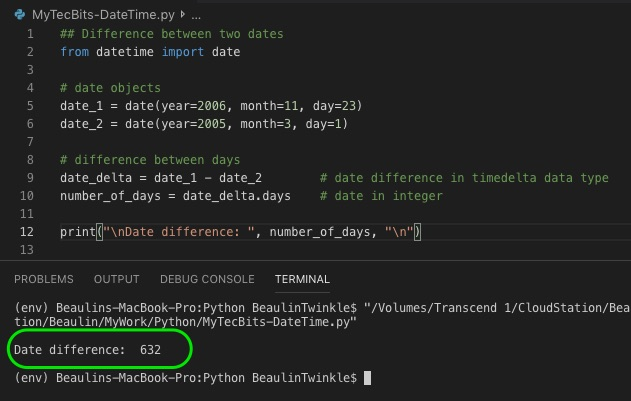# Finding days between two dates in Python

In Python, it is so simple to find the days between two dates in python. All you have to do is to subtract the starting date with the final date. The result will be a timedelta object. If you want just the number of days between dwo dates as an integer value, then you can use the timedelta object’s days attribute, to get the days in integer. Here is an example.

```## Difference between two dates
from datetime import date

# date objects
date_1 = date(year=2006, month=11, day=23)
date_2 = date(year=2005, month=3, day=1)

# difference between days
date_delta = date_1 - date_2        # date difference in timedelta data type
number_of_days = date_delta.days    # days in integer

print("\nDate difference: ", number_of_days, "\n")
```

## Reference

• More about date object in datetime module at Python docs.

### Related Articles & Tools

This site uses Akismet to reduce spam. Learn how your comment data is processed.